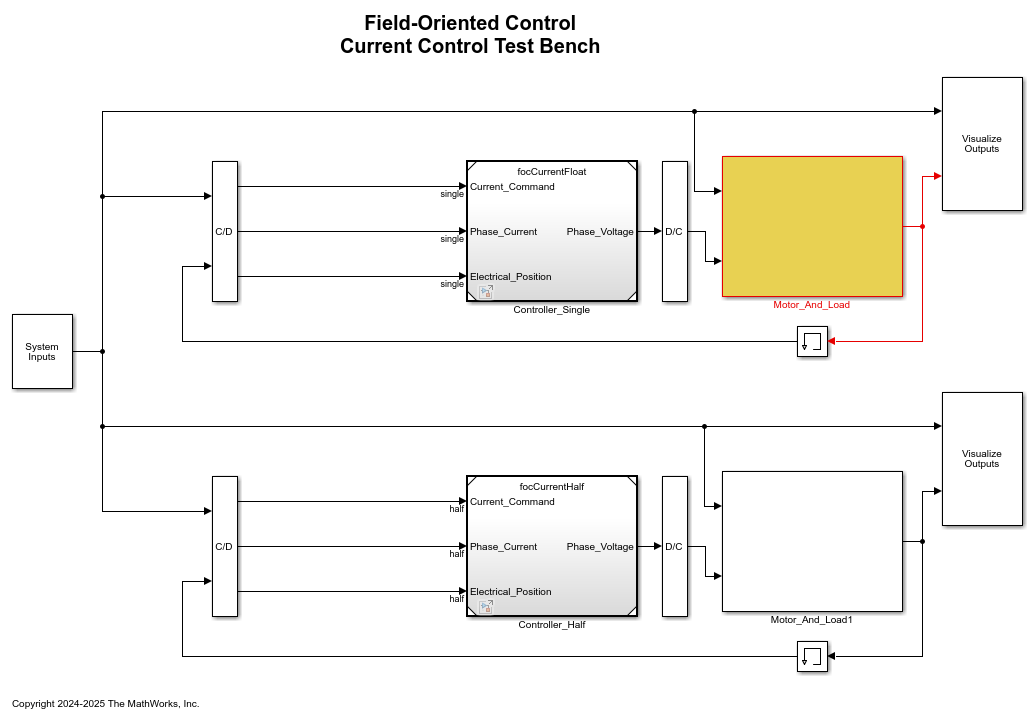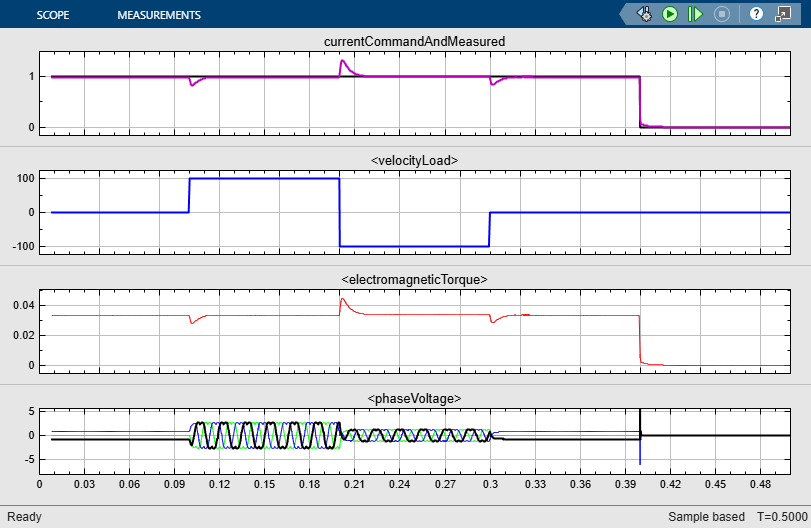# Half-Precision Field-Oriented Control Algorithm

This example shows how to implement a Field-Oriented Control (FOC) algorithm for a Permanent Magnet Synchronous Machine (PMSM). The example shows both a single-precision floating-point implementation and a half-precision floating-point implementation. When an algorithm contains large or unknown dynamic ranges (for example integrators in feedback loops) or when the algorithm uses operations that are difficult to design in fixed-point (for example, `atan2`), it can be advantageous to use floating-point representations. The half-precision data type occupies only 16 bits of memory, but its floating-point representation enables it to handle wider dynamic ranges than integer or fixed-point data types of the same size.

Load the data required to simulate the model.

```focModelData ```

Open the `ex_foc_current` model. This model uses the same source block for two versions of a field-oriented control algorithm. The first version uses single-precision data types, while the second uses half-precision data types.

```model = 'ex_foc_current.slx'; open_system(model) ```Simulate the model. You can see from the scope that the response of the single-precision implementation is identical to the response of the half-precision implementation.

```sim(model) ```
```ans = Simulink.SimulationOutput: logTestBench: [1x1 Simulink.SimulationData.Dataset] SimulationMetadata: [1x1 Simulink.SimulationMetadata] ErrorMessage: [0x0 char] ```